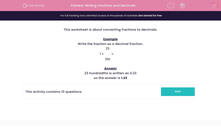# Writing Fractions and Decimals

In this worksheet, students convert fractions (including mixed numbers) into decimal fractions.Key stage:  KS 2

Curriculum topic:   Maths and Numerical Reasoning

Curriculum subtopic:   Decimals

Difficulty level:#### Worksheet Overview

This worksheet is about converting fractions to decimals.

Example

Write the fraction as a decimal fraction.

1 + 23
100
=